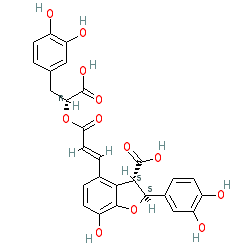=====================================================SMILES: O=C(O)[C@H](OC(/C=C/C1=C2[C@H](C(O)=O)[C@@H](C3=CC(O)=C(O)C=C3)OC2=C(O)C=C1)=O)CC4=CC(O)=C(O)C=C4
InChIKey: UJZQBMQZMKFSRV-RGKBJLTCSA-N

–EOF–